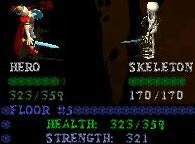-=+=- -=+=- -=+=- -=+=- -=+=- -=+=- -=+=- -=+=- -=+=- -=+=- -=+=- -=+=- -=+=- -=+=- -=+=- -=+=- -=+=- -=+=- -=+=- -=+=- -=+=- -=+=- -=+=- -=+=- -=+=- -=+=- -=+=- -=+=- -=+=- -=+=- (c) WidthPadding Industries 1987 0|693|0 -=+=- -=+=- -=+=- -=+=- -=+=- -=+=- -=+=- -=+=- -=+=- -=+=- -=+=- -=+=- -=+=- -=+=- -=+=- -=+=- -=+=- -=+=- -=+=- -=+=- -=+=- -=+=- -=+=- -=+=- -=+=- -=+=- -=+=- -=+=- -=+=- -=+=-
SoCoder -> Showcase Home -> Role Playing

curtasticCreated : 18 August 2017
Edited : 18 August 2017
System : Windows
Language : Blitz Max

### Dungeon Runes

A text-RPG

Screenshots- A text RPG, but with graphically animated battles.
- A numbers-go-up type game where you watch your stats go up after each battle.
- Collect weapons, armor, and of course 7 types of magical runes!
- Find rare items, buy and sell in the market.
- Venture into the dungeon where each floor is harder than the last. Your goal is to get to the 20th floor to defeat the dragon.

"awesome game" - Joe W.
"genius" - Eric L.
"can get addicting for a cheap text game where you just keep pressing enter" - me

Coded in July 2005I'm glad you like it thanks for playing